## Thursday, March 31, 2016

### Place Value Math Practice #1

This math practice includes 15 examples of place value for ones, tens, hundreds, and thousands place value. Each slide has an example and asks what digit is in a particular place value. This can easily be expanded to other math practice. For example, participants can read the number out loud and identify the other digits and their place value as well.

Directions

1. Click on the first slide to enlarge.
2. Click on the slide or thumbnail at the bottom to advance to the next slide.

Thanks for visiting my Student Survive 2 Thrive blog. I hope you find this math practice to be helpful!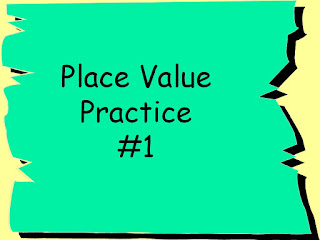Place Value Math Practice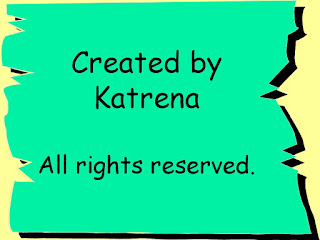Elementary Math Practice by Katrena391: Which digit is in the tens position?391: 9 is in the tens postition742: Which digit is in the ones position?742: The 2 is in the ones position508: Which digit is in the hundreds position?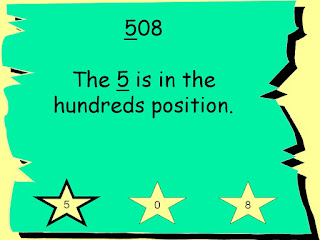508: 5 is in the hundreds position6937: Which digit is in the hundreds position?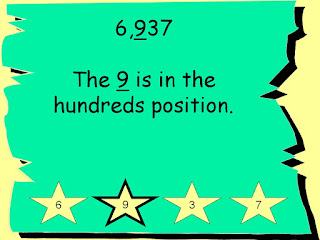6937: 9 is in the hundreds position1590: Which digit is in the ones position?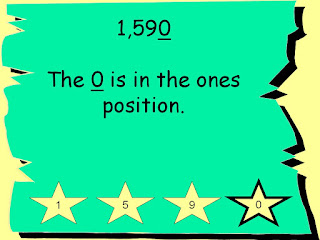1590: 0 is in the ones position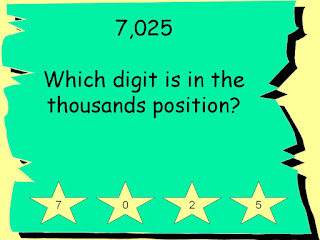7025: Which digit is in the thousands position?7025: 7 is in the thousands position4321: Which digit is in the tens position?4321: 2 is in the tens position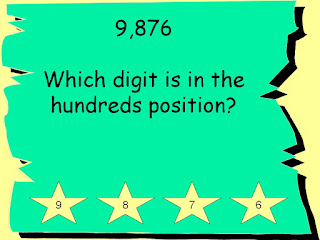9876: Which digit is in the hundreds position?9876: 8 is in the hundreds position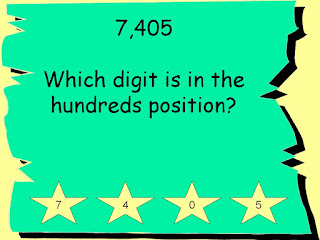7405: Which digit is in the hundreds position?7405:4 is in the hundreds position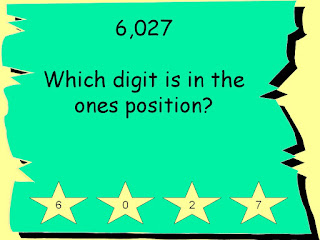6027: Which digit is in the ones position?6027: 7 is in the ones position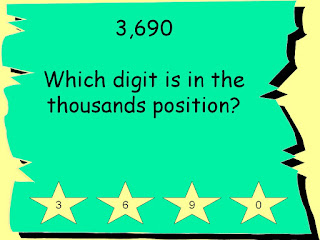3690: Which digit is in the thousands position?3690: 3 is in the thousands position8642: Which digit is in the tens position?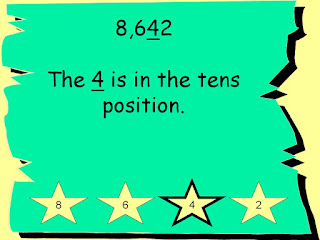8642: 4 is in the tens position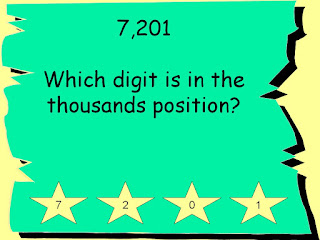7201: Which digit is in the thousands position?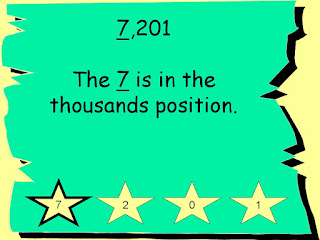7201: 7 is in the thousands position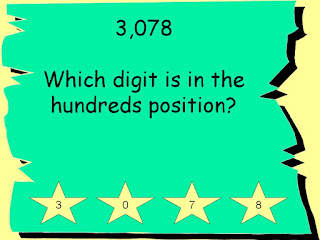3078: Which digit is in the hundreds position?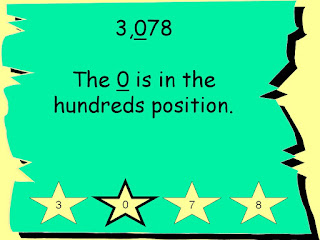3078: 0 is in the hundreds position2936: Which digit is in the ones position?4936: 6 is in the ones positionFree Elementary Math Practice on Student Survive 2 Thrive